Paul's Online Notes
Home / Calculus I / Applications of Integrals / Volumes of Solids of Revolution / Method of Rings
Show Mobile Notice Show All Notes Hide All Notes
Mobile Notice
You appear to be on a device with a "narrow" screen width (i.e. you are probably on a mobile phone). Due to the nature of the mathematics on this site it is best views in landscape mode. If your device is not in landscape mode many of the equations will run off the side of your device (should be able to scroll to see them) and some of the menu items will be cut off due to the narrow screen width.

### Section 6.3 : Volume With Rings

1. Use the method of disks/rings to determine the volume of the solid obtained by rotating the region bounded by $$y = \sqrt x$$, $$y = 3$$ and the $$y$$-axis about the $$y$$-axis.

Show All Steps Hide All Steps

Start Solution

We need to start the problem somewhere so let’s start “simple”.

Knowing what the bounded region looks like will definitely help for most of these types of problems since we need to know how all the curves relate to each other when we go to set up the area formula and we’ll need limits for the integral which the graph will often help with.

Here is a sketch of the bounded region with the axis of rotation shown.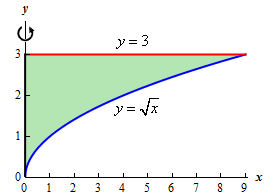Hint : Give a good attempt at sketching what the solid of revolution looks like and sketch in a representative ring.

Note that this can be a difficult thing to do especially if you aren’t a very visual person. However, having a representative disk can be of great help when we go to write down the area formula. Also, getting the representative disk can be difficult without a sketch of the solid of revolution. So, do the best you can at getting these sketches.

Show Step 2

Here is a sketch of the solid of revolution.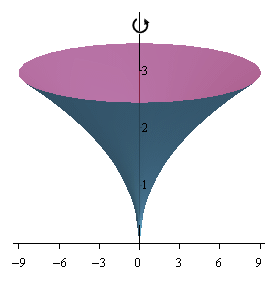Here are a couple of sketches of a representative disk. The image on the left shows a representative disk with the front half of the solid cut away and the image on the right shows a representative disk with a “wire frame” of the back half of the solid (i.e. the curves representing the edges of the of the back half of the solid).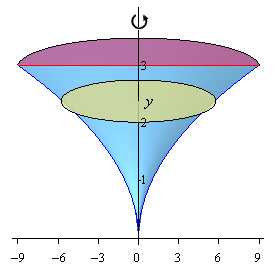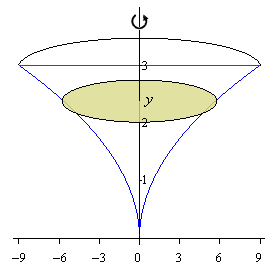Hint : Determine a formula for the area of the ring.
Show Step 3

We now need to find a formula for the area of the disk. Because we are using disks that are centered on the $$y$$-axis we know that the area formula will need to be in terms of $$y$$. This in turn means that we’ll need to rewrite the equation of the boundary curve to get into terms of $$y$$.

Here is another sketch of a representative disk with all of the various quantities we need put into it.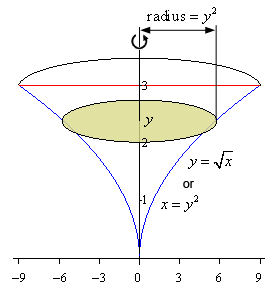As we can see from the sketch the disk is centered on the $$y$$-axis and placed at some $$y$$. The radius of the disk is the distance from the $$y$$-axis to the curve defining the edge of the solid. In other words,

${\mbox{Radius }} = {y^2}$

The area of the disk is then,

$A\left( y \right) = \pi {\left( {{\mbox{Radius}}} \right)^2} = \pi {\left( {{y^2}} \right)^2} = \pi {y^4}$ Show Step 4

The final step is to then set up the integral for the volume and evaluate it.

For the limits on the integral we can see that the “first” disk in the solid would occur at $$y = 0$$ and the “last” disk would occur at $$y = 3$$. Our limits are then : $$0 \le y \le 3$$.

The volume is then,

$V = \int_{0}^{3}{{\pi {y^4}\,dy}} = \left. {\frac{1}{5}\pi {y^5}} \right|_0^3 = \require{bbox} \bbox[2pt,border:1px solid black]{{\frac{{243}}{5}\pi }}$